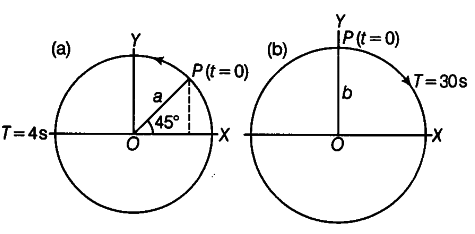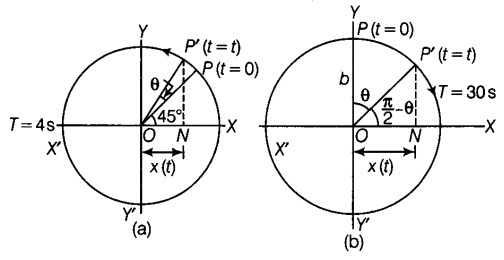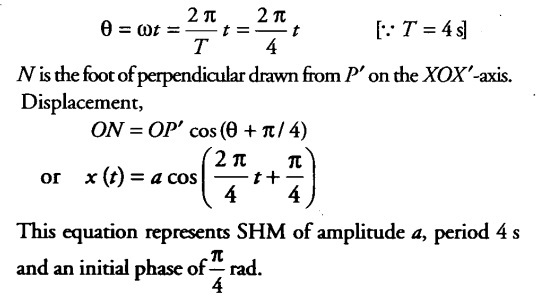# The following figures depict two circular motions

The following figures depict two circular motions. The radius of the circle, the period of revolution, the initial position and the sense of revolution are indicated on the figures.
Obtain the simple harmonic motion of the x-projection of the radius vector of the rotating particle P in each case.In figure, suppose the particle moves in the anti-clockwise sense from P to P’ in time t as shown in Fig. (a).
Angle swept by the radius vector,